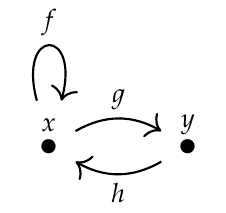Robert wrote:

> Which sequences of natural numbers arise by counting the number of paths of length n from the chosen node in a pointed graph to itself?

> I'll consider a simpler graph: one that has distinct loops of lengths \$$a_1, ... a_k\$$. Then the number of paths with length \$$n\$$ that start and end at the chosen node is the number of ways to pick nonnegative integer coefficients \$$b_i\$$ such that:

> $n=\sum_i^k a_i \cdot b_i$

> I have no idea how to simplify that expression, but I claim it to be the answer to John's first question.

There are two issues here:

1. how you are reducing the case of a general graph to the simpler sort of graph you're discussing, and

2. how your formula works for those simpler graphs.

I'll focus on point 2. Could you illustrate how your formula works if I start with the "Fibonacci graph"?It seems to be of your simpler sort, with a loop of length 1 and a loop of length 2.